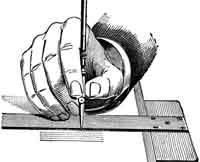#Difference Between Similar Terms and Objects

# Difference Between Dilution and Dilution FactorDilution vs Dilution Factor

In chemistry, there are terminologies and processes that chemists should consider. This not only applies to chemistry but also other bodies of knowledge and disciplines requiring mixing of chemicals for industrial productions or product applications requiring the right amount of chemicals.

Two words that will be presented and differentiated are “dilution” and “dilution factor.” Let us tackle and see the difference of the two terminologies.
Dilution has been considered to be done by early scientists during early civilizations. Dilution is the process of diluting or mixing two or more substances or even compounds. In scientific laboratories, some chemical solutions can be more concentrated than the desired solution. This is a dilemma when a protocol is being given and followed. Thus, knowledge about dilution and the formula must be mastered before venturing into this kind of career. Dilutions are expressed in exponential form. Dilution is also a term for reducing the concentration of a formula.

Dilution factor or DF, on the other hand, is a term used to describe the ratio of the final volume over the aliquot volume. To get the final volume, you just have to add the aliquot volume plus diluents. To clearly understand this process, aliquot is defined as the sub-volume of the original solution or sample. Diluent, on the other hand, is the material or substance in which the solution or sample was diluted.
For example, what will be the diluents factor if a chemist adds 0.2 ml of aliquot to 10.8 ml of diluent?

a)Let us get the final volume (aliquot volume + diluent).

Final volume = 0.2 ml + 10.8 ml.

Final volume = 10.4 ml.

b) Then for diluent factor (final volume / aliquot volume)

Diluent factor = 10.4 ml / 0.2 ml

Diluent factor = 1:52 dilution (101)
Thus, in this question, the diluents factor is 52 given by the formula. These topics are very important among companies, particularly industrial companies, who are producers of materials and products for human usage and consumption. Thus, this is an important concept for them to apply.

Summary:

1.Dilution is a process with no formula while a diluents factor requires a formula to get the answer.
2.Dilution is the process of reducing the amount of concentration while the diluents factor is the ratio of the final volume over aliquot volume.

### Search DifferenceBetween.net :

Custom Search

Help us improve. Rate this post!Loading...Email This Post : If you like this article or our site. Please spread the word. Share it with your friends/family.